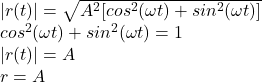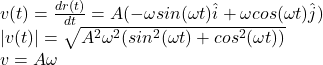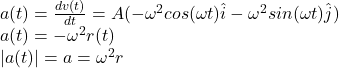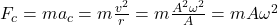## The position of a particle is given i(t) = A(coswt i + sin wt 1), where w is a constant. (a) Show that the particle moves in a circle of rad

Question

The position of a particle is given i(t) = A(coswt i + sin wt 1), where w is a constant. (a) Show that the particle moves in a circle of radius A. (b) Calculate and then show that the speed of the particle is a constanta (c) Determine and show that a is given by ae = rw?. (d) Calculate the centripetal force on the particle.

in progress 0
3 months 2021-08-13T21:03:35+00:00 1 Answers 0 views 0

Explanation:

(a) we can prove that the particle moves in a circle by taking the square of the norm of r(t)the norm of the position vector does not depend of time, so |r| is constant and is a radius of a circle.

(b) the sped of the particle is the norm of the velocity v(t). Velocity is calculated by derivating r(t)A and w are constant, hence the speed of the particle is constant.

(c) the acceleration is the derivative of the velocity(d)I hope this is useful for you

regards